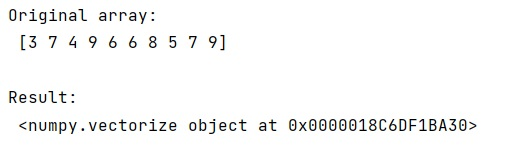# Using NumPy Vectorize on Functions that Return Vectors

Learn, how to use numpy vectorize on function so that it returns vectors?
Submitted by Pranit Sharma, on February 14, 2023

NumPy is an abbreviated form of Numerical Python. It is used for different types of scientific operations in python. Numpy is a vast library in python which is used for almost every kind of scientific or mathematical operation. It is itself an array which is a collection of various methods and functions for processing the arrays.

The numpy.vectorize() takes a function f:a->b and turns it into g:a[]->b[]. This works fine when a and b are scalars, but I can't think of a reason why it wouldn't work with b as a ndarray.

The purpose of numpy.vectorize() is to transform functions which are not numpy-aware (e.g. take floats as input and return floats as output) into functions that can operate on (and return) numpy arrays.

There is a parameter called signature which will solve our problem.

Let us understand with the help of an example,

## Python code to demonstrate the example of using numpy vectorize on functions that return vectors

```# Import numpy
import numpy as np

# Creating a numpy array
arr = np.array([3,7,4,9,6,6,8,5,7,9])

# Display original array
print("Original array:\n",arr,"\n")

# Defining a function
def fun(x):
return x * np.array([1,1,1,1,1],dtype=np.float32)

# Vectorizing function
res = np.vectorize(fun,signature='()->(n)')

# Display result
print("Result:\n",res)
```

Output: